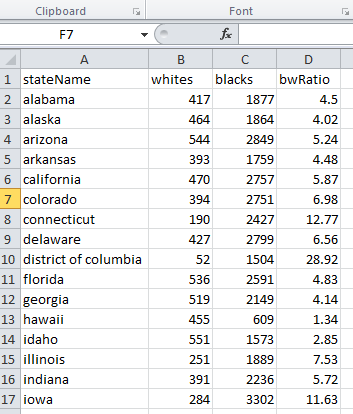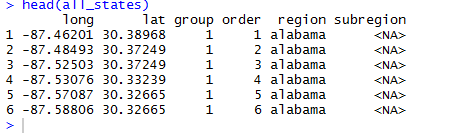Want to share your content on R-bloggers? click here if you have a blog, or here if you don't.Today’s short post will show how to make a simple map using map_data().

Let’s assume you have data in a CSV file that may look like this:Notice the lower case state names; they will make merging the data much easier. The variable of interest we’re going to plot is the relative incarceration rates by race (whites and blacks) across each of the fifty states (we’ll remove DC once we load the data). Using the map_data(“state”) command, we can load a data.frame called “all_states”, shown below:Merging that data with the data frame we have as a CSV produces:We can then plot each state and shade it by our variable of interest:Full code is below: